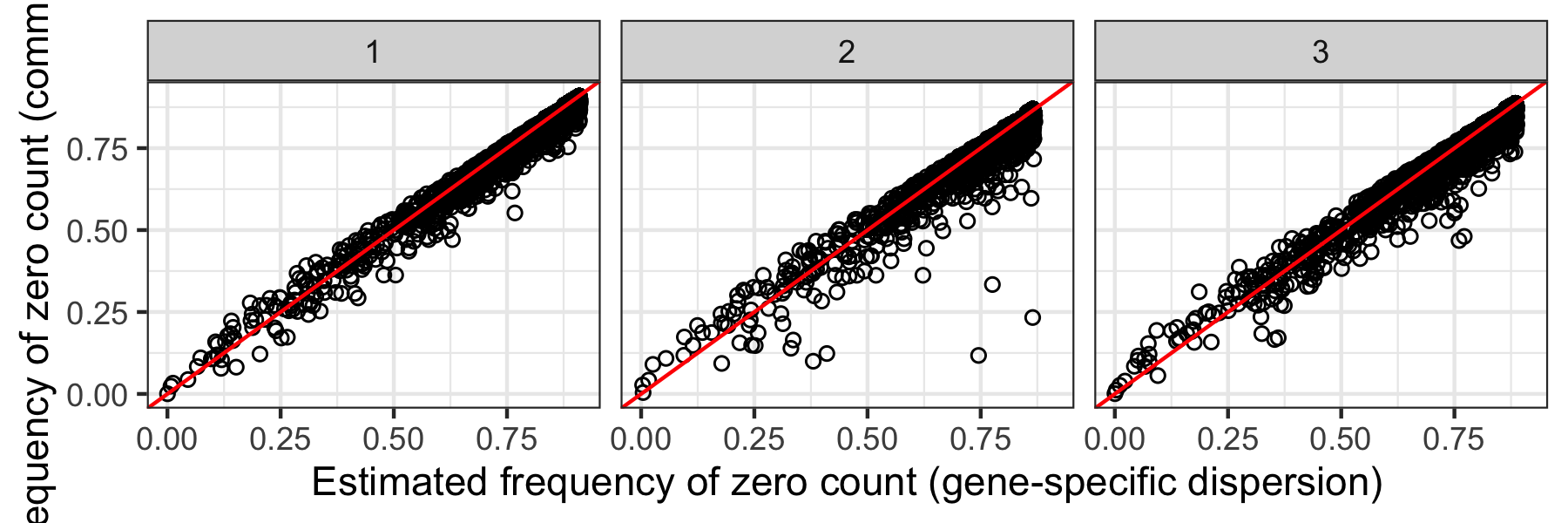Introduction to PhitestR

2022-01-20

PhitestR currently supports input of a UMI count matrix with cluster labels.

To demonstrate the usage of PhitestR with a count matrix, we use an example dataset which can be downloaded from this link. To use PhitestR, we need a count matrix (in this example, object) with rows representing genes and columns representing cells. In addition, we also need a (character or numeric) vector of labels (in this case, label) specifying the assignments of the cells.

object = data$count label = data$labels

Now, to run Phitest, we just need to specify the count matrix, cluster labels, and the number of cores to be used in parallel computation. The result is a list containing two elements. The first element is a vector of P values corresponding to the clusters in label.

result = phitest(object, label, ncores = 2)
result$pval The second element is a named list of estimated parameters corresponding to the clusters. The list names are the cluster labels, and each element is a matrix with rows representing genes and columns representing estimated parameters. For example, we could make similar plots as shown in the paper with the code below: library(ggplot2) labels = names(result$par)
par = lapply(labels, function(l){
tp = result$par[[l]] tp$cluster = l
return(tp)
})
par = Reduce(rbind, par)
ggplot(par, aes(x = dhat, y = dhat.c)) +
geom_point(shape = 1, cex = .5) +
geom_abline(intercept = 0, slope = 1, color = "red") +
facet_wrap(~cluster) +
xlab("Estimated frequency of zero count (gene-specific dispersion)") +
ylab("Estimated frequency of zero count (common dispersion)")Estimated parameters of Phitest by clusters.